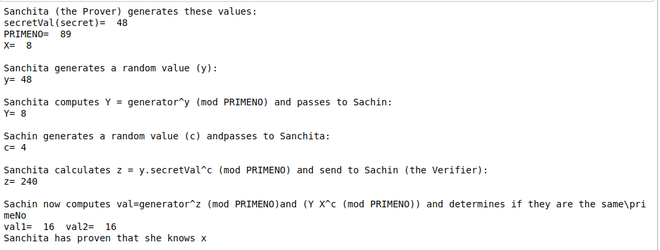# Schnorr Identification Scheme

Schnorr signature is known for its simplicity and is among the first whose security is based on the intractability of certain discrete logarithm problems. The Schnorr digital signature scheme is different from the identification scheme. An identification scheme is used for the holder of the private key to prove to you that they hold the private key. A signature scheme in addition proves that the key holder used their private key with a specific message as an input value in order to generate a verifiable digital signature on that specific message.

## Schnorr Identification Scheme with the discrete logarithm

Meaning of equivalence ‘≡’

The elements of the integers modulo (often shortened to “mod”) 36 are 0, 1, . . . , 34, 35. Then 41 is equivalent to 5 modulo 36, written 41 ≡ 5 mod 36, because when 41 is divided by 36 the remainder is 5.

Similarly, we also have 57 ≡ 5 mod 26.

Discrete logarithm:

Discrete logarithms are logarithms defined with regard to multiplicative cyclic groups. If G is a multiplicative cyclic group and g is a generator of G, then from the definition of cyclic groups, we know every element h in G can be written as gx for some x. The discrete logarithm to the base g of h in the group G is defined to be x .

If the group is A, and the generator is 2, then the discrete logarithm of 1 is 4 because 24 ≡ 1 mod 5.

Logarithm logb(a) is a number x such that bx = a, for given numbers a and b. Analogously, in any group G, powers bk can be defined for all integers k, and the discrete logarithm logb(a) is an integer k such that bk = a.

We have the following 3 parameters:

• g is the Generator,
• x is the secret value
• p is the prime number.

Algorithm

The prover Sanchita has a proving public key of (g, X) where g is a generator, and X=gx(modN). After Sanchita generates her public proving key, she will then be challenged by Sachin to produce the correct result. A discrete logarithm is an integer x satisfying the equation ax≡b(modm) where a and m are relatively prime.

With Schnorr identification, Sanchita (the prover) has a proving public key of (N, g, X) and a proving secret key of (N, x). N is a prime number for the modulus operation, and x is the secret, and where:

```X←gx(modN)
```

On the registration of the secret, Sanchita generates a random value (y), and then computes Y:

```Y←gy(modN)
```

This value is sent to Sachin (who is the verifier). Sachin then generates a random value (c) and sends this to Sanchita. This is a challenge for Sanchita to produce the correct result. Sanchita then computes:

```z←(y+xc)(modN)
```

He then sends this to Sachin in order to prove that he knows x. Sachin then computes two values:

```val1 = YXc(modN)
val2 = gz(modN)
```

If the values are the same (val1≡val2), Sanchita has proven that she knows x.

This works because:

```YXc = gygxc = gy+cx
gz = gy+cx
```

## Python3

 `import` `random ` `import` `sys ` ` `  `PRIMENO ``=` `89` `generator ``=` `3` ` `  ` `  `secretVal ``=` `random.randint(``1``, ``97``) ` ` `  `X ``=` `pow``(generator, secretVal) ``%` `PRIMENO ` `y ``=` `random.randint(``1``, ``97``) ` `Y ``=` `pow``(generator, y) ``%` `PRIMENO ` ` `  `print``(``"Sanchita (the Prover) generates these values:"``) ` `print``(``"secretVal(secret)= "``, secretVal) ` `print``(``"PRIMENO= "``, PRIMENO) ` `print``(``"X= "``, X) ` ` `  `print``(``"\nSanchita generates a random value (y):"``) ` `print``(``"y="``, y) ` ` `  `print``("\nSanchita computes Y ``=` `generator^y \ ` `(mod PRIMENO) ``and` `passes to Sachin:") ` ` `  `print``(``"Y="``, Y) ` ` `  `print``("\nSachin generates a random value (c) ``and``\ ` `passes to Sanchita:") ` ` `  `c ``=` `random.randint(``1``, ``97``) ` `print``(``"c="``, c) ` `print``("\nSanchita calculates z ``=` `y.secretVal^c \ ` `(mod PRIMENO) ``and` `send to Sachin (the Verifier):") ` ` `  `z ``=` `(y ``+` `c ``*` `secretVal) ` ` `  `print``(``"z="``, z) ` ` `  `print``("\nSachin now computes val``=``generator^z (mod PRIMENO)\ ` `and` `(Y X^c (mod PRIMENO)) ``and` `determines ``if` `they are the same\primeNo") ` ` `  `val1 ``=` `pow``(generator, z) ``%` `PRIMENO ` `val2 ``=` `(Y ``*` `(X``*``*``c)) ``%` `PRIMENO ` ` `  `print``(``"val1= "``, val1, end``=``' '``) ` `print``(``" val2= "``, val2) ` ` `  `if` `(val1 ``=``=` `val2): ` `    ``print``(``"Sanchita has proven that she knows x"``) ` `else``: ` `    ``print``(``"Failure to prove"``) `

Output:Application:

• It has its use case in cryptographic research
• It is used in the implementation of Zero-Knowledge Proof.
• It provides a more secure way for authentication without the prover revealing its secret key.

My Personal Notes arrow_drop_upCheck out this Author's contributed articles.

If you like GeeksforGeeks and would like to contribute, you can also write an article using contribute.geeksforgeeks.org or mail your article to contribute@geeksforgeeks.org. See your article appearing on the GeeksforGeeks main page and help other Geeks.

Please Improve this article if you find anything incorrect by clicking on the "Improve Article" button below.

Article Tags :
Practice Tags :

Be the First to upvote.

Please write to us at contribute@geeksforgeeks.org to report any issue with the above content.